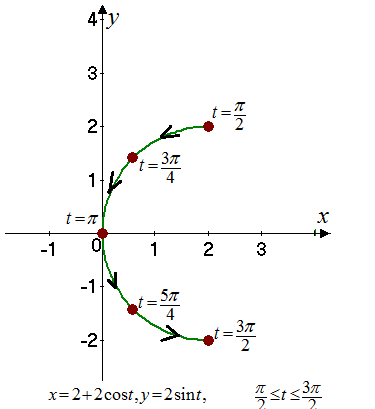# The parametric equation for the particle that moves counter clockwise halfway around the circle from the top and bottom.### Single Variable Calculus: Concepts...

4th Edition
James Stewart
Publisher: Cengage Learning
ISBN: 9781337687805### Single Variable Calculus: Concepts...

4th Edition
James Stewart
Publisher: Cengage Learning
ISBN: 9781337687805

#### Solutions

Chapter 1, Problem 32RE

(a)

To determine

## To find: The parametric equation for the particle that moves counter clockwise halfway around the circle from the top and bottom.

Expert Solution

The parametric equation is x=2+2cost , y=2sint for the parameter range of π2t3π2 .

### Explanation of Solution

Given:

The given equation is (x2)2+y2=4 .

Calculation:

The general form for the parametric equation of the circle with the centre at (h,k) and radius r is,

x=h+rcosty=k+rsint

The range of t is,

0t2π

Consider the given equation of the circle is,

(x2)2+y2=4

Then, the parametric equation is,

x=2+2costy=2sint

As the path of the particle is in counter clockwise direction from the top to the bottom, the value of t varies from π2 to 3π2 . Thus, the parameter range is,

π2t3π2

The parametric equation is x=2+2cost , y=2sint for the parameter range of π2t3π2 .

(b)

To determine

Expert Solution

### Explanation of Solution

Given:

The given equation is (x2)2+y2=4 .

Calculation:

Consider the parametric equation are,

x=2+2costy=2sint

Consider the parameter range is,

π2t3π2

The table for the value of x and y for different values of the parameter is shown in Table 1

Table 1

 t x y π2 2 2 3π4 0.5858 1.414 π 0 0 5π4 0.5858 -1.414 3π2 2 -2

The graph for the parametric equations is shown in Figure 1Figure 1

### Have a homework question?

Subscribe to bartleby learn! Ask subject matter experts 30 homework questions each month. Plus, you’ll have access to millions of step-by-step textbook answers!